0
2205

# RRB JE Reasoning Questions PDF

Download RRB JE Arithmetic Questions and Answers PDF. Top 25 RRB JE Reasoning questions based on asked questions in previous exam papers very important for the Railway JE exam.Question 1: A piece of paper is folded and punched as shown below in the question figures. From the given answer figures indicate how will the figure be opened?

a)

b)

c)

d)

Question 2: Find the figure which will complete the given figure

a)

b)

c)

d)

Question 3: A figure is given below. Choose the option in which the question figure is inscribed.

a)

b)

c)

d)Question 4: Choose the figure which will come next in the series.

a)

b)

c)

d)

Question 5: Which figure among the option must logically replace the ‘?’ in the diagram below –

a)

b)

c)

d)

Question 6: Three of the four figures are alike in a certain way and hence form a group. Which of the following doesn’t belong to the group?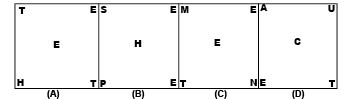a) A

b) B

c) C

d) D

Question 7: Three of the four figures given in the options are alike in a certain way and hence form a group. Which of the following doesn’t belong to that group?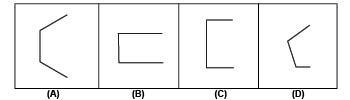a) A

b) B

c) C

d) D

Question 8: Study the figures shown in the options below. Three of them are alike in a certain way and hence form a group. Identify the one that doesn’t belong to that group?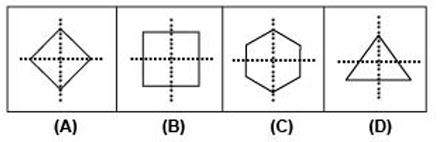a) A

b) B

c) C

d) D

Question 9: Find the missing number?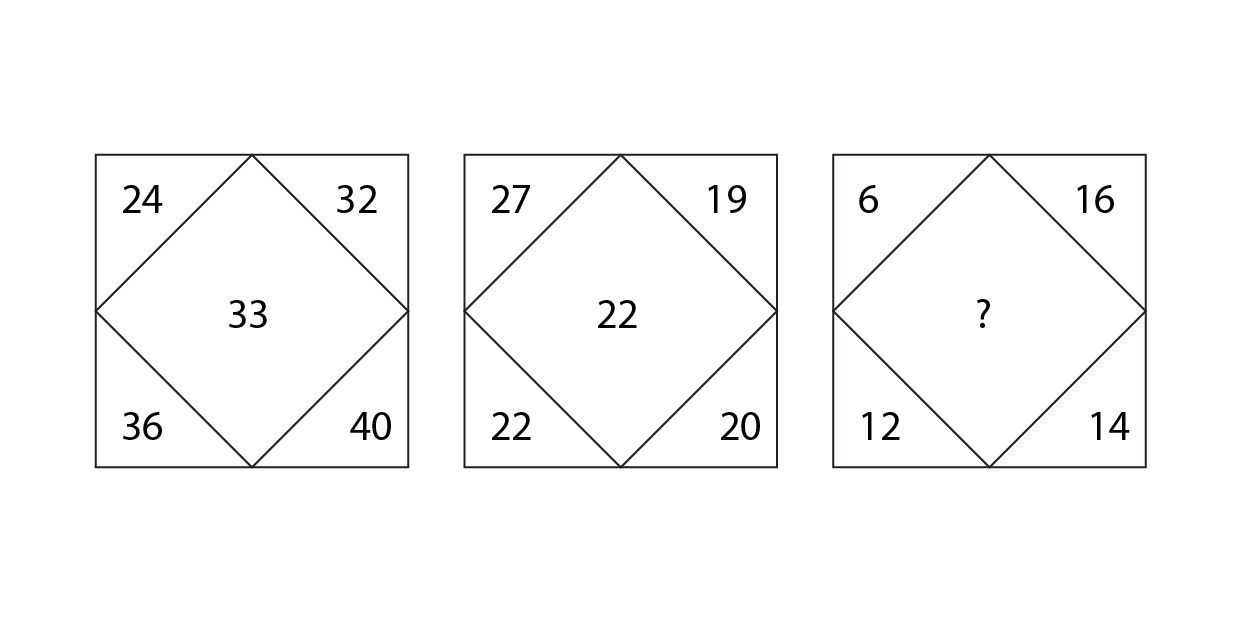a) 10

b) 12

c) 14

d) 11Question 10: How many squares are there in this figure?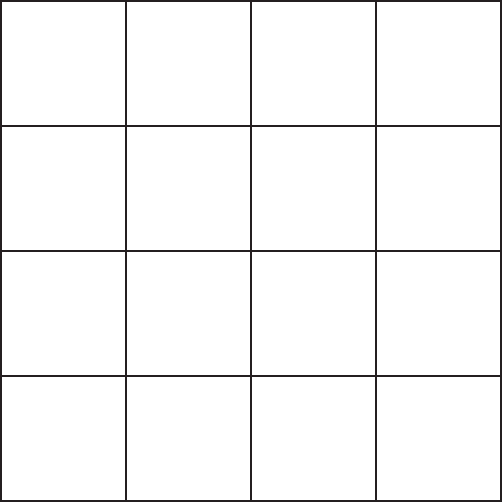a) 16

b) 27

c) 29

d) 30

Question 11: Find the missing number?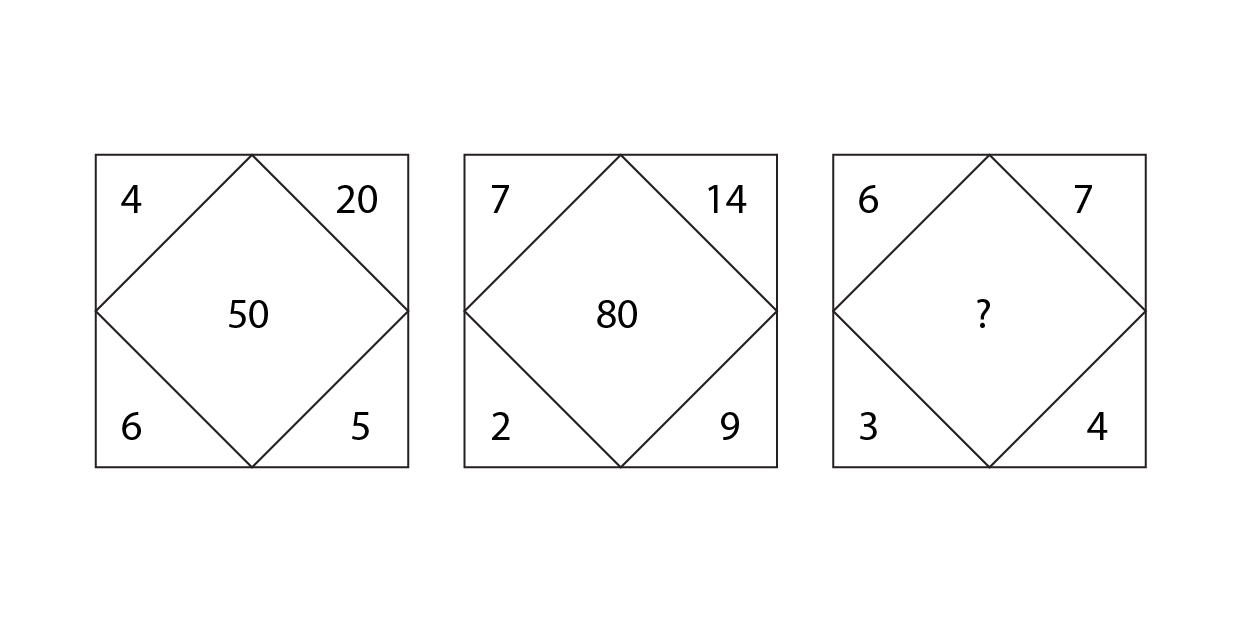a) 30

b) 40

c) 50

d) 70

Question 12: A standard dice is as shown. What is the number on the bottom face?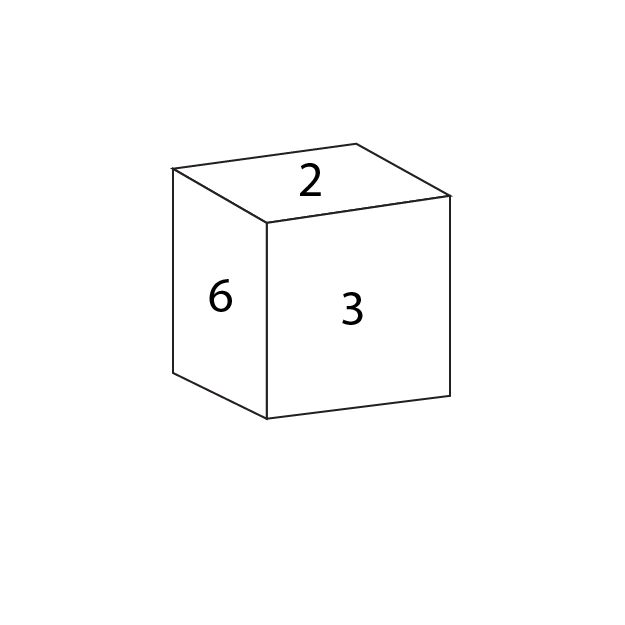a) 1

b) 4

c) 5

d) Can’t say

Instructions

If A%B means “A is the mother of B”
A@B means “A is the father of B”
A+B means “A is the brother of B”
A$B means “A is the sister of B” A#B means “A is the wife of B” Question 13: If J$K@L#M is true, how is M related to J’s brother?

a) Brother-in-law

b) Daughter-in-law

c) Son-in-law

d) Niece

e) Cannot be determined

Question 14: If R@Q+O$P is true, how is R related to P? a) Father b) Daughter c) Cousin d) Son e) Cannot be determinedQuestion 15: If M#N+K%Z is true, how is Z related to N? a) Cousin b) Niece c) Nephew d) Aunt e) Cannot be determined Question 16: Looking at a photograph, P (male) said, ‘His father’s mother’s husband’s only daughter’s husband is my only sister’s uncle’ How is the man in the photograph of related to P? a) The photograph is of P himself b) Father c) Cousin d) Brother e) Cannot be determined Question 17: if’ AxB’ means B is the father of A and ‘A+B’ means A is the wife of B and A /B means A is the brother of B , then what is the relation of J with L in the ‘ J + H / R x L ‘. a) Daughter b) Daughter in law c) Sister in law d) Cannot determined e) None of these Instructions In the following question three statements are given, followed by two conclusions. You have to consider the statements to be true even if they seem to be at variance from commonly known facts. You have to decide which of the given conclusions, if any, follows from the given statements and select the appropriate option. Question 18: Statement I: All pens are markers. Statement II: No pen is a pencil. Statement III: Some pencils are markers. Conclusion I: All pencils can be markers. Conclusion II: All markers can be pencils. a) Conclusion I only b) Conclusion II only c) Both the conclusions follow d) Either conclusion I or conclusion II follows e) None of the conclusions follows Question 19: Statement I: Some chairs are stools. Statement II: No stool is a table. Statement III: All dusters are tables. Conclusion I: Some dusters can be chairs. Conclusion II: All tables can be chairs. a) Conclusion I only b) Conclusion II only c) Both the conclusions follow d) Either conclusion I or conclusion II follows e) None of the conclusions follows Question 20: Statement I: Some triangles are pentagons. Statement II: All pentagons are circles. Statement III: No circle is a hexagon. Conclusion I: No circle is a triangle. Conclusion II: Some triangles can be hexagons. a) Conclusion I only b) Conclusion II only c) Both the conclusions follow d) Either conclusion I or conclusion II follows e) None of the conclusions followsQuestion 21: What will come next in the following series? 2, 12, 36, 80, 150, 252, ? a) 325 b) 400 c) 392 d) 484 e) 296 Question 22: In a certain language, ‘He likes singing’ is coded as 254, ‘Singing is boring’ is coded as 682, ‘Singing songs’ is coded as 92, ‘She likes dancing’ is coded as ‘715’. Which of the following can be the code for ‘Dancing likes boring songs’? a) 8596 b) 1857 c) 5896 d) 5678 e) 6159 Question 23: If A * CC *CD = CEEC, where A, C, D, and E are single digit whole numbers, what is the value of A+C-D+E? a) 11 b) 5 c) 8 d) 10 e) 7 Question 24: If the code for SUPER is 32145, the code for PROM is 8259 and the code for SUDI is 0743, then which of the following can be the code for PRIDE? a) 05892 b) 71205 c) 20578 d) 73591 e) 35170 Instructions Rajesh starts from his office and drives 12 Km north to reach a temple. He takes a right turn and drives 8 Km to reach a grocery store. He turns left and drives 3 km and then takes a right turn and drives 4 Km to reach a pizza shop. He collects the pizza, takes a right turn and drives 5 km to reach a crossroad, from which he takes a left turn and drives 12 Km to reach his house. There is a tennis court to the north of the grocery store such that the pizza shop is equidistant to both the grocery store and the tennis court. Question 25: What is the shortest distance between his office and his house? a)$10\sqrt{5}$Km b) 26 Km c)$20\sqrt{2}$Km d) 25 Km e)$8\sqrt{5}$Km Answers & Solutions: 1) Answer (B) The paper is folded twice so the punched shape must be replicated four times. After opening the figure, we get, Hence, option B is right choice. 2) Answer (A) We can see that sum at the center is in AP. Hence, the sum in the missing figure must be 28 + 3 = 31. Hence, it could be either option A or D. However, option D is not symmetric. Hence, option A is the answer. 3) Answer (C) Option A misses out the pentagon which is present in the given figure. Option B has the pentagon but misses out on the triangle which is inscribed in the pentagon. Option D suffers from a similar flaw. Option C best inscribes the given figure. Hence, B is the correct answer. 4) Answer (A) The number of triangles is increasing by 1 in each subsequent figure. Hence, the next figure will have 5 triangles. Thus, option A is the correct answer. 5) Answer (B) We see that new symbol is added everytime at the start and the earlier symbols move along the sides of the square in clockwise direction. Thus we get, Hence, option B is right choice. 6) Answer (C) All the figures are comprised of meaningful English words except (c). Figure A can be rearranged into TEETH. Figure B can be rearranged into SHEEP. Figure C can be rearranged into MEENT. Figure D can be rearranged into ACUTE. 7) Answer (B) In the given options, we can observe that all the figures have regular polygons cut into half. (a) is half of a regular hexagon (b) is half of a rectangle (c) is half of a regular quadrilateral (square). (d) is an half of a pentagon, which is also a regular polygon. 8) Answer (D) All the figures shown are symmetrical over the X axis except (d). 9) Answer (B) The number in the center is the average of all the numbers in the corners. Hence, the missing number = (6+16+12+14) /4= 48/4=12 10) Answer (D) There are 16 squares of 1 unit. There are 9 squares of 4 units. There are 4 squares of 9 units and there is 1 square of 16 units. Hence, there are 16+9+4+1=30 squares. 11) Answer (A) The number in the center is the product of the top two numbers minus the product of the bottom two numbers. Hence, the missing number=42-12=30. 12) Answer (C) Opposite faces of a dice add up to 7. Hence, the bottom face=7-2= 5. 13) Answer (C) Drawing the family tree, M is the son-in-law of J’s brother. 14) Answer (A) Drawing the family tree, R is the father of P.15) Answer (E) Drawing the family tree, Z’s gender cannot be determined. 16) Answer (E) Sister’s uncle will be P’s uncle as well. Father’s mother’s husband’s only daughter implies his aunt. However, we don’t know if his father is only son of his parents. Hence, the photograph may be of P or it may be of his cousin. e nce we cannot accurately determine whose photo is it. Hence, option E is the correct answer. 17) Answer (B) Expression : J + H / R x L J is the wife of H, and H is the brother of R. Also, L is the father of R, => H is the son of L. Thus, J is the daughter-in-law of L. => Ans – (B) 18) Answer (A) From the diagram, we can see that all pencils can be markers hence conclusion I follows. No pen is a pencil and all pens are markers. Hence, all markers can’t be pencils. Thus, conclusion II doesn’t follow. Therefore, option A is the correct answer. 19) Answer (C) From the diagram, we can see that some dusters can be chairs. Hence, conclusion I follows. From the diagram, we can see that all tables can be chairs. Hence, conclusion II follows. Therefore, option C is the correct answer. 20) Answer (B) From the diagram, we can see that some circle can be triangles. Hence, conclusion I doesn’t follow. From the diagram, we can see that some triangles can be hexagons. Hence, conclusion II follows. Therefore, option B is the correct answer. 21) Answer (C) T1 = 2 =$1^2 + 1^3$T2 = 12 =$2^2 + 2^3$T3 = 36 =$3^2 + 3^3$T4 = 80 =$4^2 + 4^3$T5 = 150 =$5^2 + 5^3$T6 = 252 =$6^2 + 6^3$So, T7 =$7^2 + 7^3$= 49 + 343 = 392 Hence, option C is the correct answer. 22) Answer (E) He likes singing – 254 Singing is boring – 682 Singing songs – 92 She likes dancing – 573 From the first 3 statements, we can infer that the code for ‘singing’ is 2 and songs is 9. From the first and the last sentences, we can infer that the code for ‘likes’ is 5. The code for ‘is’ and ‘boring’ is 6 and 8 in any order. The code for ‘she’ and ‘dancing’ is 7 and 1 in any order. Therefore, the code must contain 5, 9, exactly one among 6 and 8 and exactly one among 7 and 1. Only option E satisfies this condition. Therefore, option E is the right answer. 23) Answer (B) As we can see, the last digit is only influenced by A*C*D. A*C*D = C. Therefore, A*D must yield 1 as the last digit. Both A and D cannot be 1 or 9. 7 and 3 is the only possible combination. The product of 3 numbers is of the form CEEC. Let us check whether the number is divisible by 11. C+E -(C+E) = 0. Therefore, CEEC is divisible by 11. CC should be one among 11, 22, 33, 44, ….99. Let us start checking from 11. If CC = 11, then A*11*1D = 1EE1. A and D can be 3 and 7 in any order. If A = 3 and D = 7, then 3*11*17 = 561. 561 is not of the form 1EE1. Therefore, we can eliminate this case. If A = 7 and D = 3, then 7*11*13 = 1001. Therefore, A must be 7, D must be 3, C must be 1 and E must be 0. A + C – D + E = 7 + 1 – 3 + 0 = 5. Therefore, option B is the right answer. 24) Answer (B) SUPER and SUDI have SU in common thus SU is coded 43 and thus, DI is coded 07 and PER is coded 521. Thus, the code for PRIDE can be any combination of 52107. Among the given options only option B uses all of 52107. Hence, option B is the correct answer. 25) Answer (B) Let’s represent the given information in a diagram, The tennis court and grocery shop are equidistant from the Pizza shop and the tennis court is to the north of the grocery shop. Hence, the tennis court should be at a distance of 6 Km from the grocery shop. Now, the distance between the office and the house =$\sqrt{24^2 + 10^2}\$ = 26 KmWe hope this Reasoning Questions for RRB JE Exam will be highly useful for your preparation.